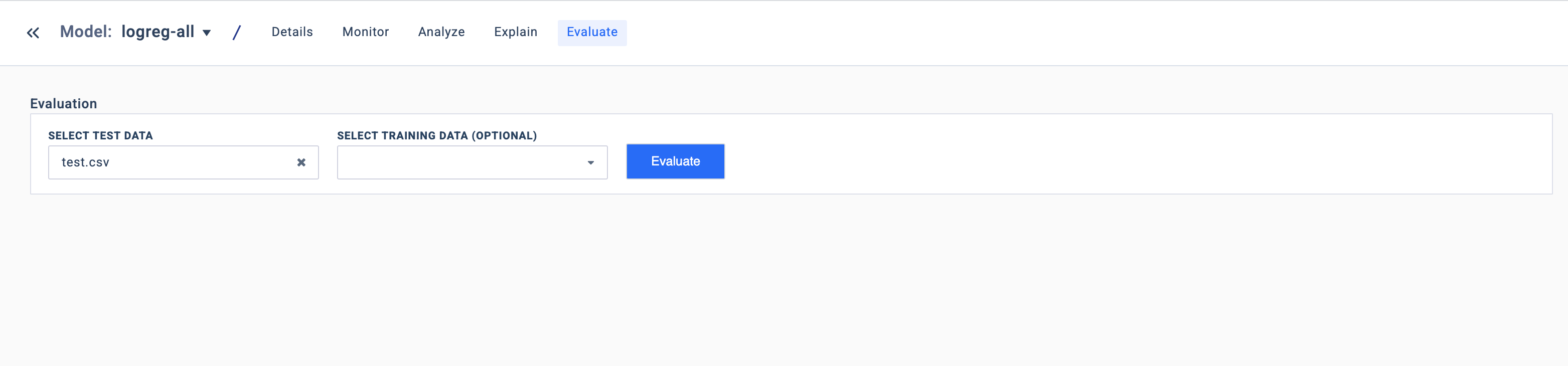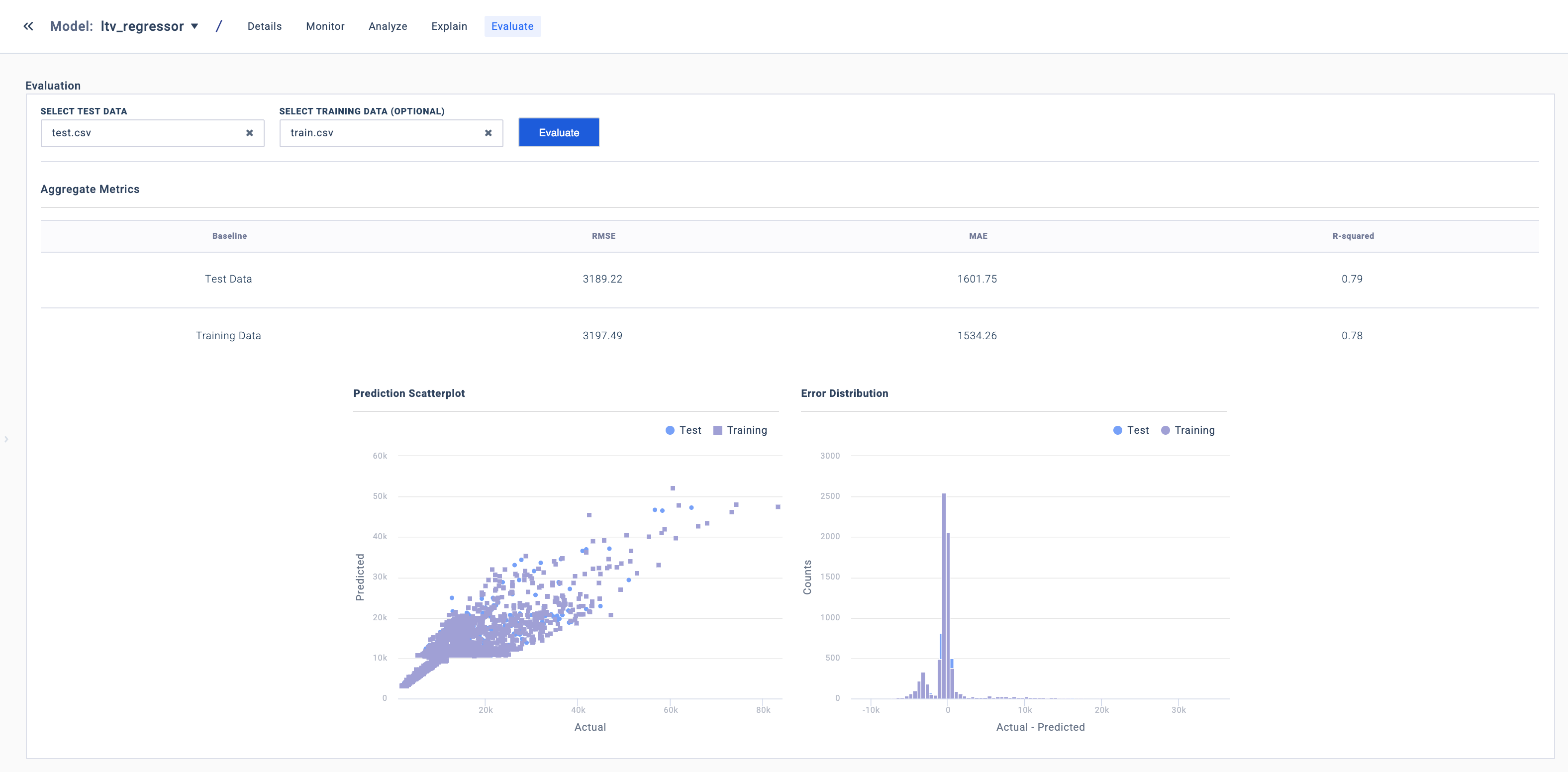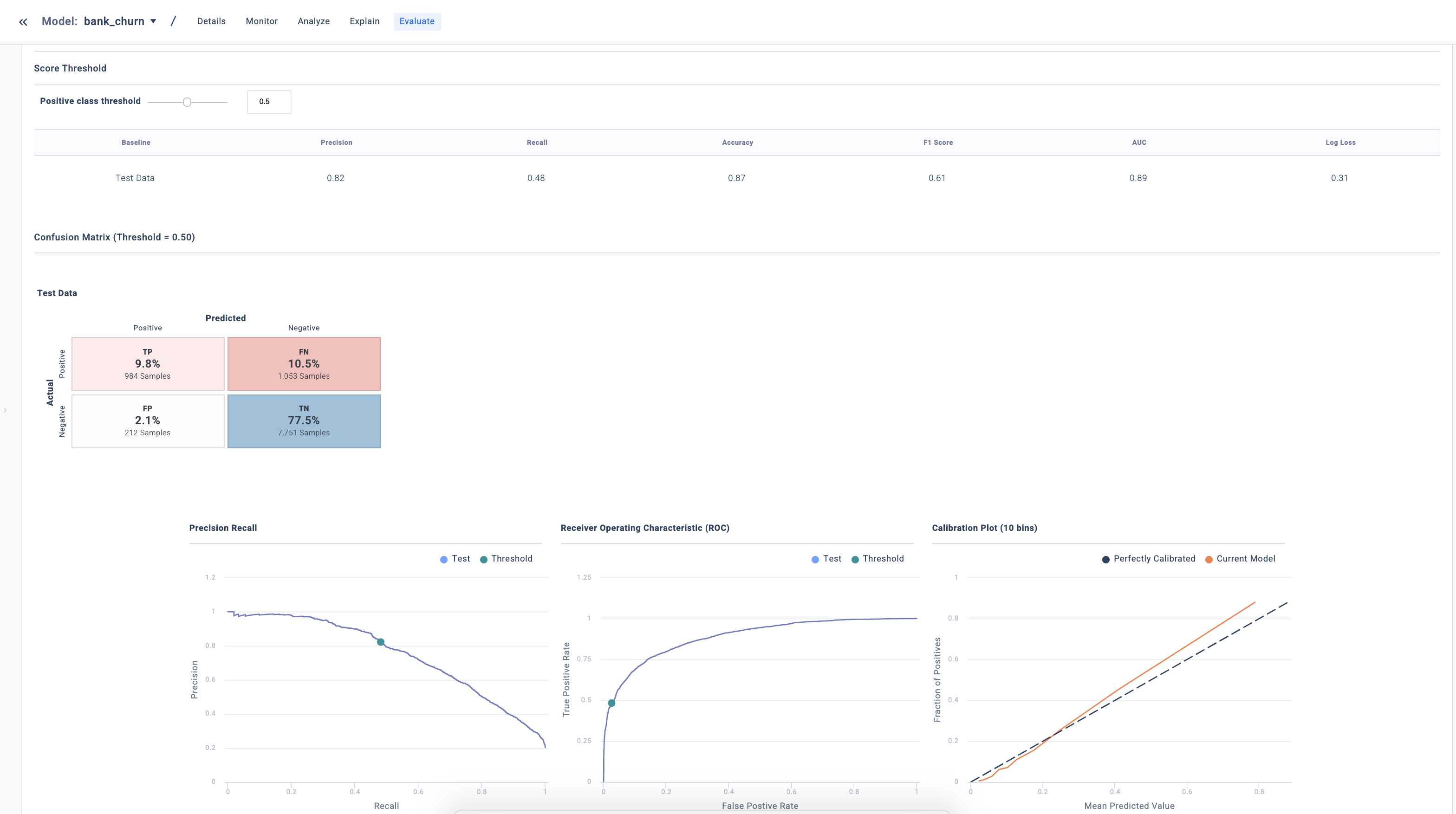# Evaluation

Model performance evaluation is one of the key tasks in the ML model lifecycle. A model's performance indicates how successful the model is at making useful predictions on data.

Once your trained model is loaded into Fiddler, click on Evaluate to see its performance.## Regression Models

To measure model performance for regression tasks, we provide some useful performance metrics and tools.• Root Mean Square Error (RMSE)
• Measures the variation between the predicted and the actual value.
• RMSE = SQRT[Sum of all observation (predicted value - actual value)^2/number of observations]
• Mean Absolute Error (MAE)
• Measures the average magnitude of the error in a set of predictions, without considering their direction.
• MAE = Sum of all observation[Abs(predicted value - actual value)]/number of observations
• Coefficient of Determination (R2)
• Measures how much better the model's predictions are than just predicting a single value for all examples.
• R2 = variance explained by the model / total variance
• Prediction Scatterplot
• Plots the predicted values against the actual values. The more closely the plot hugs the y=x line, the better the fit of the model.
• Error Distribution
• A histogram showing the distribution of errors (differences between model predictions and actuals). The closer to 0 the errors are, the better the fit of the model.

## Classification Models

To measure model performance for classification tasks, we provide some useful performance metrics and tools.• Precision
• Measures the proportion of positive predictions which were correctly classified.
• Recall
• Measures the proportion of positive examples which were correctly classified.
• Accuracy
• Measures the proportion of all examples which were correctly classified.
• F1-Score
• Measures the harmonic mean of precision and recall.
• AUC
• Measures the area under the Receiver Operating Characteristic (ROC) curve.
• Log Loss
• Measures the performance of a classification model where the prediction input is a probability value between 0 and 1. The goal of the ML model is to minimize this value.
• Confusion Matrix
• A table that shows how many predicted and actual values exist for different classes. Also referred as an error matrix.
• Receiver Operating Characteristic (ROC) Curve
• A graph showing the performance of a classification model at different classification thresholds. Plots the true positive rate (TPR), also known as recall, against the false positive rate (FPR).
• Precision-Recall Curve
• A graph that plots the precision against the recall for different classification thresholds.
• Calibration Plot
• A graph that tell us how well the model is calibrated. The plot is obtained by dividing the predictions into 10 quantile buckets (0-10th percentile, 10-20th percentile, etc.). The average predicted probability is plotted against the true observed probability for that set of points.